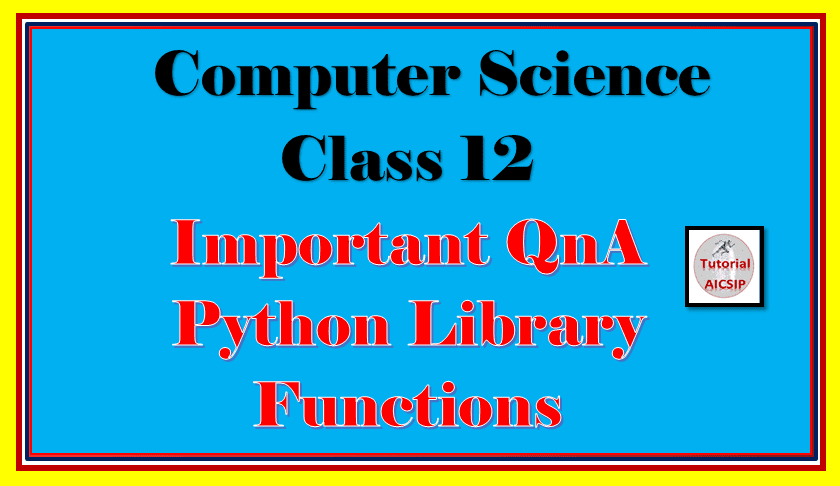In this article important QnA Python Library Functions class 12 we will discuss questions and answers for the topic Python library functions.

Topics Covered

## QnA Python Library Functions class 12

Let’s begin the article QnA Python Library Functions class 12 with theory-based questions.

## Theory Questions

1. What is a library?
2. Write a few examples of commonly used libraries with examples.
3. What is the python module? Explain the structure of the python module.
4. How to import the python module?

5. Explain the commonly used python mathematical methods/functions with examples.

6. Explain the commonly used python string/text methods/functions with examples.

Follow this link to find the above answers of QnA Python Library Functions Class 12.

In the next section of QnA Python Library Functions class 12 we will discuss some application based questions.

## Application Based questions

### Error Based questions

1. def python_lib():
x = float(input(“Enter the number:”))
y = Math.ceil((x)
Assume that, x = 10.66777878

Ans.: There are three errors in the code, import math statement is missing and y = math.ceil(x) should be used.

2. x = 5
y = math.pow(3)

Ans.: There are two errors in the code, import math statement is missing and pow() function required two parameters,

3. a = 10
b =  sqrt(a,2)
Assume that math module is imported.

Ans.: sqrt() function requires only one parameter.

4. s = “Welcome to My Blog”
print(str.s.capitalise())

Ans.: Error in print() function. The method capitalize() not written correctly and str word is not required.

### Output Questions

Note: Assume that the math module is imported wherever required.

1. x = -45.2342343254325
b = math.fabs(x)
y = math.ceil(b)
print(y+5)

Ans.: 51
fabs() function convert the nagetive value into postive. As ceil() function returns the nearest integer of the passed argument value as well as in print() function y+5 is written. So math.ceil(x) returns 👉 46, 46  + 5 = 51.

2. import math
def python_lib():
s = “Computer Science with Python”
print(s.swapcase())
print(s.title())
print(s.split())
print(s.replace(‘with’,’-‘))
python_lib()

Ans.:
cOMPUTER sCIENCE WITH pYTHON
Computer Science With Python
[‘Computer’, ‘Science’, ‘with’, ‘Python’]
Computer Science – Python

3. import math
def python_lib():
s = “Python is midlle level language. Learning python is fun.”
c = 0
w = s.split()
for i in w:
if i==’is’:
c = c + 1
print(c)
python_lib()

Ans.: 2
In this code w = s.split() is used that generates a list of words from the text. Then in the for loop, if condition is given for counting the word ‘is’ is available 2 times in the text. Then finally the counter variable is printed. So the output is 2.

4. import math
def python_lib():
s = “Python is Programing Platform that Playful”
w = s.split()
c=0
for i in w:
if i==’P’:
print(i,end=”#”)
python_lib()

Ans.: Python#Programing#Platform#Playful#
In for loop, i is searching the word starts with letter P and printed in the next line.

5.  import math
def python_lib():
s = “Python is Programing Platform that is Playful”
s1=”
print(s.lstrip(‘yPt’))
print(s.rstrip(‘Pul’))
print(s1.join([s,’ in 2020′]))
python_lib()

Ans.:
hon is Programing Platform that is Playful
Python is Programing Platform that is Playf
Python is Programing Platform that is Playful in 2020

I hope you enjoyed the article QnA Python Library Functions Class 12. If you have any doubt or query feel free to ask me in the comment section.

Than you for visit.